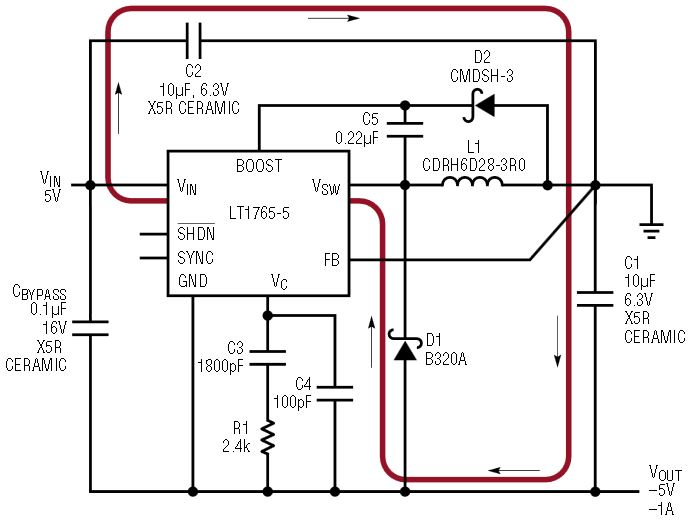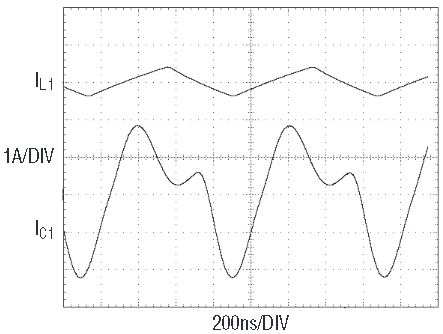# Lower the Output Voltage Ripple of Positive-to-Negative DC/DC Converters with Optimum Capacitor Hook-Up

Low ripple voltage positive-to-negative DC/DC converters are used in many of today’s high frequency and noise sensitive disc drives, battery powered devices, portable computers, and automotive applications. A positive-to-negative converter can have very low output ripple voltage (similar to a typical buck converter) as long as the bulk input capacitor is placed between VIN and VOUT, as opposed to placing it between VIN and ground. There is a common misconception that positive-to-negative converters in the former configuration have noisy outputs, but this configuration actually solves noise problems rather than introducing them. In either configuration (as shown in Figures 1a and 1b) the VIN and GND pins of an LT1765 are connected to VIN and VOUT respectively. Therefore, placing the input capacitor between VIN and VOUT is equivalent to placing it between the LT1765’s VIN and GND pins (as shown in Figure 1a). The other, commonly accepted method of placing the bulk input capacitor between VIN and ground (as shown in Figure 1b) significantly increases the output voltage ripple (see Figures 2a and 2b). To make matters worse, this configuration requires an additional high-frequency bypass capacitor between the VIN and GND pins of the IC.Figure 1a. LT1765 5V to –5V converter with bulk input cap between VIN and VOUT (IC GND pin) has low output ripple. The high dI/dt path, indicated here with bold red lines, does not include the output capacitor.Figure 1b. LT1765 5V to –5V converter with the bulk cap between VIN and ground has much higher output ripple than the circuit in Figure 1a. The high dI/dt path, indicated here with bold red lines, includes the output capacitor, thus increasing output ripple.Figure 2a. In the circuit shown in Figure 1a, the output capacitor (C1) peak-to-peak current ripple is equal to inductor peak-to-peak ripple with 1A output.Figure 2b. In the circuit shown in Figure 1b, the output capacitor (C1) peak-to-peak current ripple is five times as high as inductor peak-to-peak ripple (and therefore five times as high as the current ripple shown in Figure 2a) with 1A output.

In simple positive-to-negative converters, like those shown in Figures 1a and 1b, the output voltage ripple is:

Low ESR output capacitors, such as ceramics, help to minimize the output voltage ripple in DC/DC converters. For a given output capacitor ESR, output voltage ripple can be further reduced by minimizing the current ripple that the output capacitor is forced to absorb. In Figure 1b, the output capacitor is part of the high dI/dt switching current path, making the output voltage ripple proportionately larger.

With the bulk input capacitor placed as shown in Figure 1a, the peak-to-peak ripple current in the output capacitor is equal to the peak-to-peak ripple current in the inductor, which is designed to be relatively low for continuous-mode operation.

When the bulk input capacitor is placed as shown in Figure 1b, the peak-to-peak ripple current in the output capacitor is much higher than the inductor’s ripple current alone; it is almost equal to the inductor’s ripple current plus the input capacitor’s ripple current.

With much lower output capacitor ripple current, the size of the output capacitor in the circuit shown in Figure 1a can be much smaller than that of the circuit shown in 1b. Also, it does not need to handle nearly as much RMS ripple current (approximately equal to peak-to-peak ripple current divided by the square root of twelve).

Another advantage of removing the output capacitor from the high dI/dt switching loop (by judicious placement of the input capacitor) is that the layout is greatly simplified. The high dI/dt components shown in Figure 1 must be placed in the smallest loop possible to minimize trace inductance and the resulting voltage (noise) spikes. With one less component to worry about in the layout, it is easier to create a noise-free circuit using the layout shown in Figure 1a than it is using the one shown in Figure 1b.

Conclusion

Instead of placing the bulk input capacitor between the input supply and ground, place it across the input and ground pins of the step-down converter IC such as the LT1765. The result is significantly lower voltage ripple at the output and a simpler circuit design.